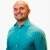# How to apply the long division algorithm divide two polynomialsThis lesson will look at an example question involving polynomials. This lesson will give a step by step explanation of how to apply the long division algorithm divide two polynomials and to better explain the concept.
0 Views